TL;DR: This is an informal summary of our recent paper Blended Matching Pursuit with Cyrille W. Combettes, showing that the blending approach that we used earlier for conditional gradients can be carried over also to the Matching Pursuit setting, resulting in a new and very fast algorithm for minimizing convex functions over linear spaces while maintaining sparsity close to full orthogonal projection approaches such as Orthogonal Matching Pursuit.

## What is the paper about and why you might care

We are interested in solving the following convex optimization problem. Let $f$ be a smooth convex function with potentially additional properties and $D \subseteq \RR^n$ a finite set of vectors. We want to solve:

$\tag{opt} \min_{x \in \operatorname{lin}D} f(x)$

The set $D$ in the context considered here is often referred to as dictionary and its elements are called atoms. Note that this problem does also make sense for infinite dictionaries and more general Hilbert spaces but for the sake of exposition we confine ourselves here to the finite case; see the paper for more details.

### Sparse Signal Recovery

The problem (opt) with, e.g., $f(x) \doteq \norm{x - y}_2^2$ for a given vector $y \in \RR^n$ is of particular interest in Signal Processing in the context of Sparse Signal Recovery, where a signal $y \in \RR^n$ is measured that is known to be the sum of a sparse linear combination of elements in $D$ and a noise term $\epsilon$, e.g., $y = x + \epsilon$ for some $x \in \operatorname{lin} D$ and $\epsilon \sim N(0,\Sigma)$; see Wikipedia for more details. Here sparsity refers to $x$ being a linear combination of few elements from $D$ and the task is to reconstruct $x$ from $y$. If the signal’s sparsity is known ahead of time, say $m$, then the optimization problem of interest is:

$\tag{sparseRecovery} \min_{x \in \RR^k} \setb{\norm{y - Dx}_2^2 \ \mid\ \norm{x}_0 \leq m},$

where $|D| = k$ and $m \ll k$ typically. As the above problem is non-convex (and in fact NP-hard to solve), various relaxations have been used and a common one is to solve (opt) instead with an algorithm that promotes sparsity due to its algorithmic design. Other variants include relaxing the $\ell_0$-norm constraint via an $\ell_1$-norm constraint and then solving the arising constrained convex optimization problem over an appropriately scaled $\ell_1$-ball with an optimization methods that is relatively sparse, such as e.g., conditional gradients and related methods.

The following graphics is taken from Wikipedia’s Matching Pursuit entry. On the bottom the actual signal is depicted in the time domain and on top the inner product of the wavelet atom with the signal is shown as a heat map, where each pixel corresponds to a time-frequency wavelet atom (this would be our dictionary). In this example, we would seek a reconstruction with $3$ elements given by the centers of the ellipsoids.Without going into detail here, (sparseRecovery) also naturally relates to compressed sensing and our algorithm also applies to this context, as do all other algorithms that solve (sparseRecovery).

### The general setup

Here we actually consider the more general problem of minimizing an arbitrary smooth convex function $f$ over the linear span of the dictionary $D$ in (opt). This more general setup has many applications including the one from above. Basically, whenever we seek to project a vector into a linear space, writing it as linear combination of basis elements we are in the setup of (opt). Moreover, sparsity is often a natural requirement as it helps explainability and interpretation etc. in many cases.

### Solving the optimization problem

Apart from the broad applicability, (opt) is also algorithmically interesting. It is a constrained problem as we optimize subject to $x \in \operatorname{lin} D$, yet at the same time the feasible region is unbounded. Surely one could project into the linear space etc but this is quite costly if $D$ is large and potentially very challenging if $D$ is countably infinite; in fact it is (opt) that solves exactly this problem for a specific vector $y$ subject to additional constraints such as, e.g., sparsity and good Normalized Mean Squared Error (NMSE). When solving (opt) we thus face some interesting challenges, such as not being able to bound the diameter of the feasible region (an often used quantity in constrained convex minimization).

There are various algorithms to solve (opt) while maintaining sparsity. One such class are Coordinate Descent, Matching Pursuit [MZ], Orthogonal Matching Pursuit [AKGT] and similar algorithms that try to achieve sparsity due to their design. Another class solves a constraint version by introducing an $\ell_1$-constraint as discussed above to induce sparsity. This includes (vanilla) Gradient Descent (not really sparse), Conditional Gradient descent [CG] (aka the Frank-Wolfe algorithm [FW]) and its variants (see e.g., [LJ]) as well as specialized algorithms such as Compressive Sampling Matching Pursuit (CoSaMP) [NT] or Conditional Gradient with Enhancement and Truncation (CoGEnT) [RSW]. Also our recent Blended Conditional Gradients (BCG) algorithm [BPTW] applies to the formulation with $\ell_1$-ball relaxation; see also the summary of the paper for more details.

For an overview of the computational as well as reconstruction advantages and disadvantages of some of those algorithms, see [AKGT].

## Our results

More recently, in [LKTJ] a unifying view of Conditional Gradients and Matching Pursuit has been established. Apart from presenting new algorithms, the authors also show that basically the Frank-Wolfe algorithm corresponds to Matching Pursuit and the Fully-Corrective Frank-Wolfe algorithm corresponds to Orthogonal Matching Pursuit; shortly after in [LRKRSSJ] an accelerated variant of Matching Pursuit has been provided. The unified view of [LKTJ] motivated us to carry over the blending idea from [BPTW] to the Matching Pursuit context, as the BCG algorithm provided very good sparsity in the constraint case in our tests. Moreover, we wanted to extend the convergence analysis to not just smooth and (strongly) convex functions but more generally smooth and sharp functions, which nicely interpolates between the convex and the strongly convex regime (see Cheat Sheet: Hölder Error Bounds (HEB) for Conditional Gradients for details on sharpness); the same can be also done for Conditional Gradients (see our recent work [KDP] or the summary).

The basic idea behind behind blending is to mix together various types of steps. Here the mixing is between Matching Pursuit style steps and low-complexity Gradient Steps over the currently selected atoms. The former steps make sure that we discover new dictionary elements that we need to make progress, whereas the latter ones usually give more per-iteration progress, are cheaper in wall-clock time, and promote sparsity. Unfortunately, as straightforward as it sounds to carry over the blending to Matching Pursuit, it is not that simple. The blending that we did before in [BPTW] heavily relied on dual gap estimates (in fact variants of the Wolfe gap) to switch between the various steps, however these gaps are not available here due to the unboundedness of $\operatorname{lin} D$.

After navigating these technical challenges, what we ended up with is a Blended Matching Pursuit (BMP) algorithm, that is basically as fast (or faster) than the standard Matching Pursuit (or its generalized variant, Generalized Matching Pursuit (MP), for arbitrary smooth convex functions), while maintaining a sparsity close to that of the much slower Orthogonal Matching Pursuit (OMP); the former only performs line search across the newly added atom, while the latter re-optimizes over the full set of selected elements in each iteration, hence offering much better sparsity at the price of much higher running times.

### Example Computation 1:

The following figure shows a sample computation for a sparse signal recovery instance from [RSW], which we scaled down by a factor of $10$. The actual signal has a sparsity of $s = 100$, we have $m = 500$ measurements, and the measurement happens in $n = 2000$-dimensional space. We choose $A\in\mathbb{R}^{m\times n}$ and $x^\esx \in \mathbb{R}^n$ with $\norm{x^\esx}_0=s$. The measurement is generated as $y=Ax^\esx + \mathcal{N}(0,\sigma^2I_m)$ with $\sigma = 0.05$.

We benchmarked BMP against MP and OMP (see [LKTJ] for pseudo-code). We also benchmarked against BCG (see [BPTW] for pseudo-code) and CoGEnT (see [RSW] for pseudo-code); for these algorithms we optimize subject to a scaled $\ell_1$-ball, where the radius has been empirically chosen so the signal is contained in the ball; otherwise we could not compare primal gap progress. Note that by scaling up the $\ell_1$-ball we might produce less sparse solutions; see [LKTJ] and the contained discussion for relating conditional gradient methods to matching pursuit methods. Each algorithm is run for either $300$ secs or until there is no (substantial) primal improvement anymore; whichever comes first.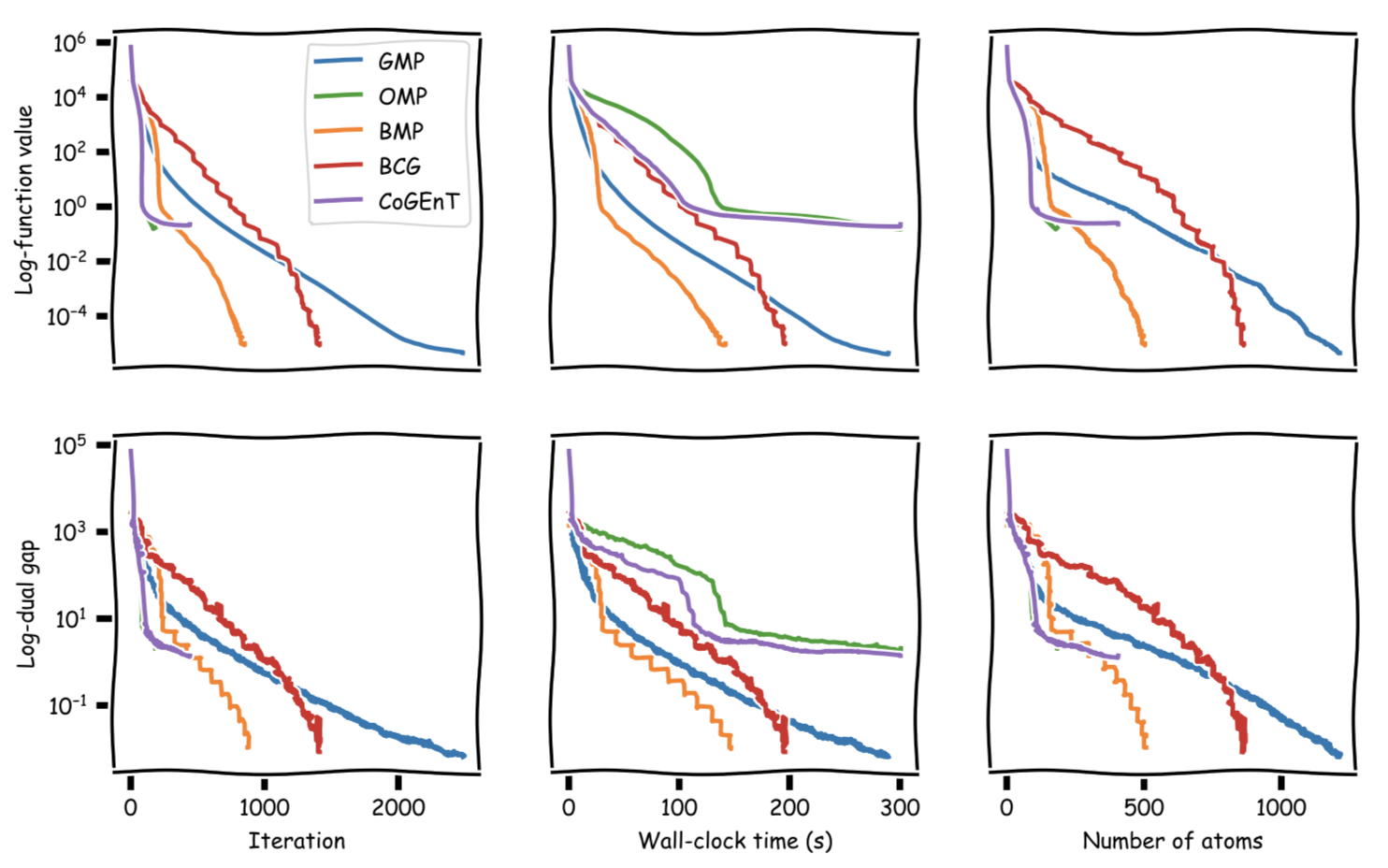In the aforementioned Sparse Signal Recovery problem, another way to compare the quality of the actual reconstructions is via the Normalized Mean Square Error (NMSE). The next figure shows the evolution of NMSE across the optimization: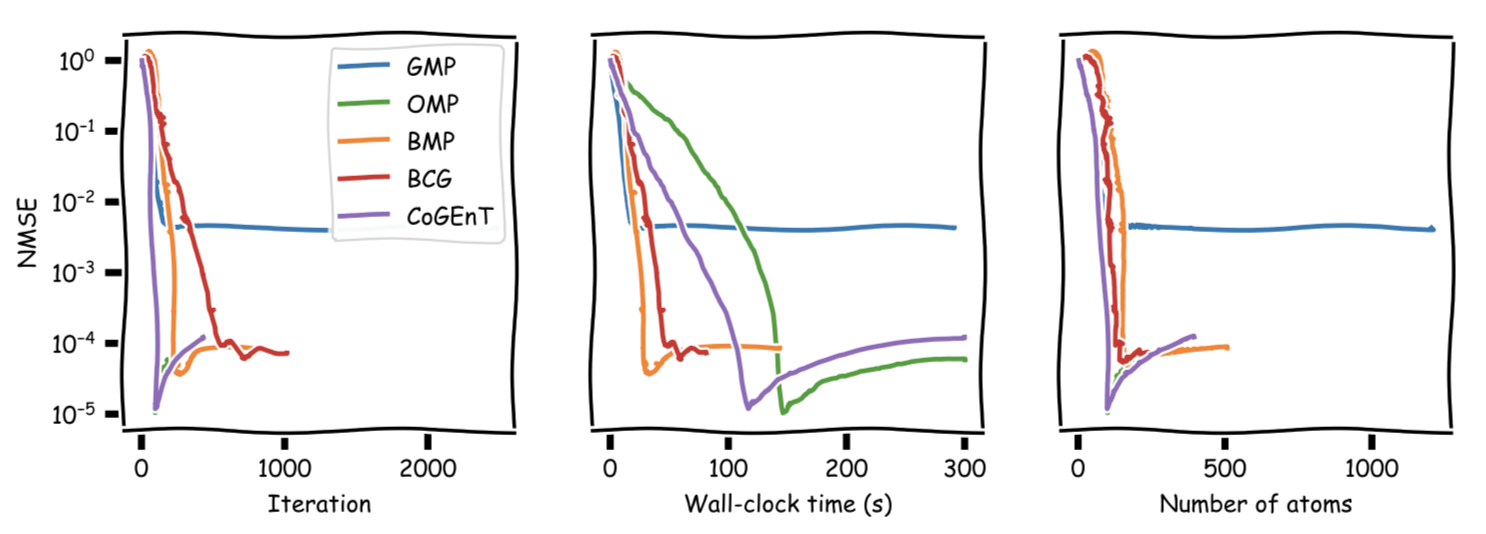The rebound likely happens because once the actual signal is reconstructed, overfitting of the noise term starts, deteriorating NMSE. One could clean up the reconstruction by removing all atoms in the support with small coefficients; this is beyond the scope however. Here the same NMSE plot truncated after the first $30$ secs for better visibility of the initial phase: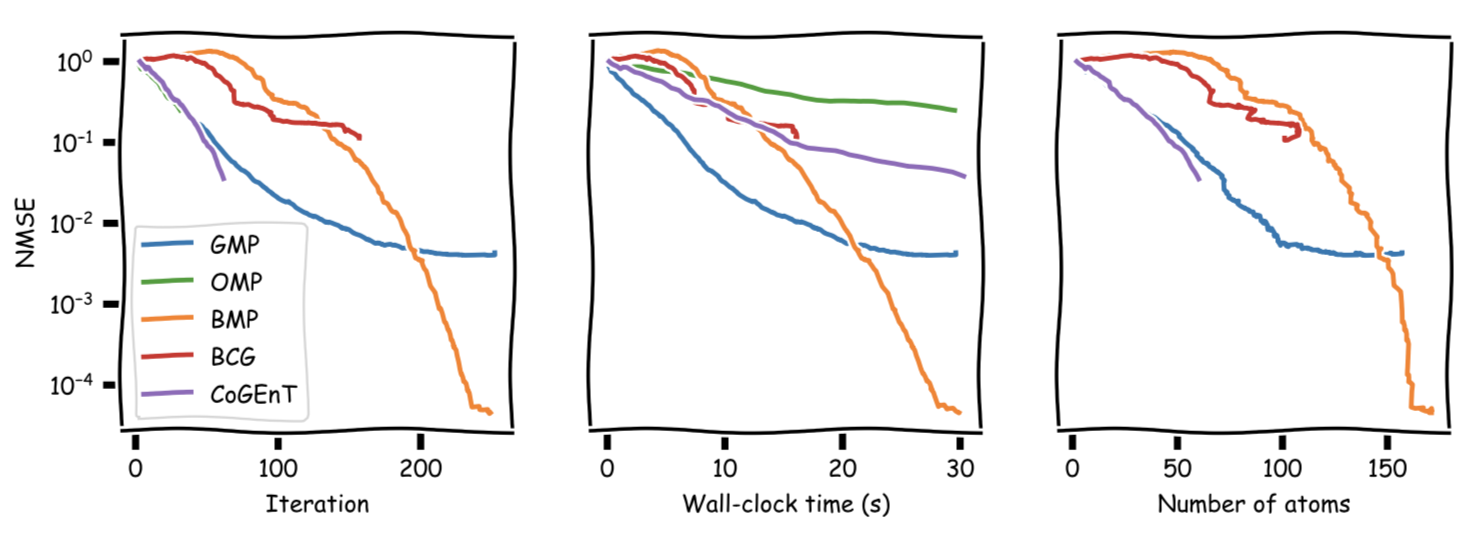### Example Computation 2:

Same setup as above, however this time actual signal has a sparsity of $s = 100$, we have $m = 1500$ measurements, and the measurement happens in $n = 6000$-dimensional space. This time we run for $1200$ secs or until no (substantial) primal progress. Here the performance of BMP is very obvious.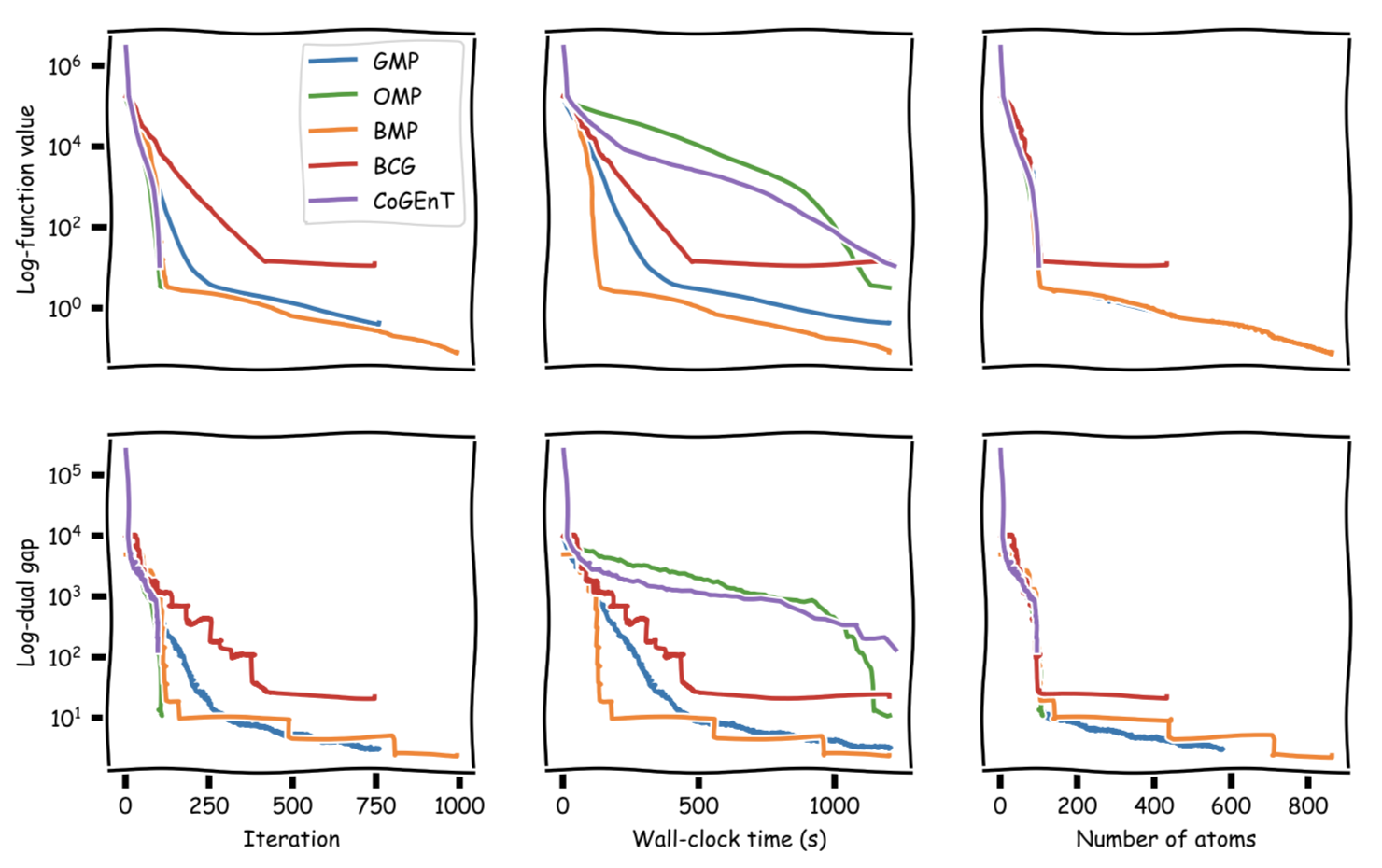The next figure shows the evolution of NMSE across the optimization: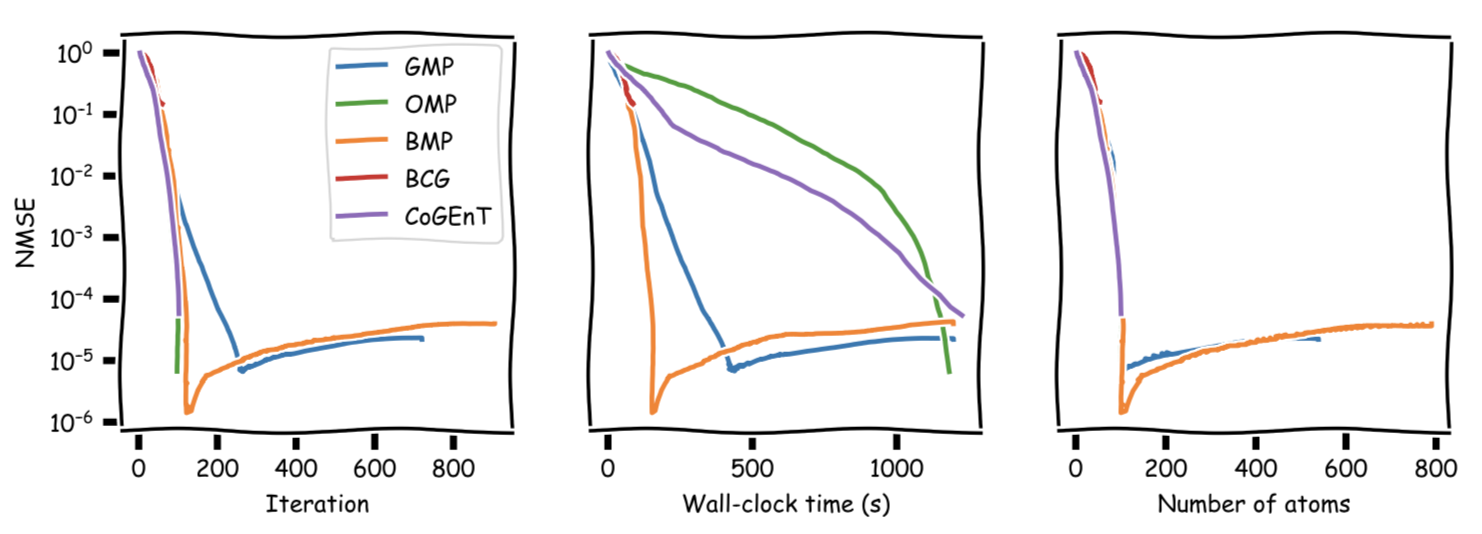And truncated again, here after roughly the first $300$ secs for better visibility. We can see that BMP reaches its NMSE minimum right around $100$ atoms and it is much faster than any of the other algorithms.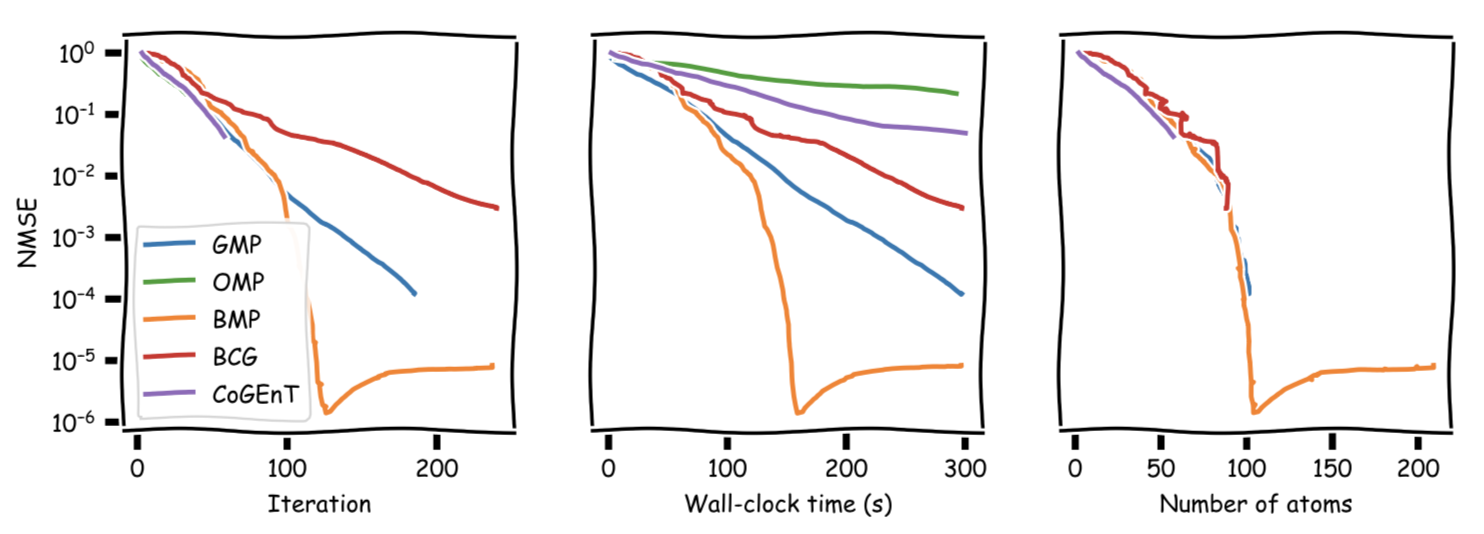[MZ] Mallat, S. G., & Zhang, Z. (1993). Matching pursuits with time-frequency dictionaries. IEEE Transactions on signal processing, 41(12), 3397-3415. pdf

[TG] Tropp, J. A., & Gilbert, A. C. (2007). Signal recovery from random measurements via orthogonal matching pursuit. IEEE Transactions on information theory, 53(12), 4655-4666. pdf

[CG] Levitin, E. S., & Polyak, B. T. (1966). Constrained minimization methods. Zhurnal Vychislitel’noi Matematiki i Matematicheskoi Fiziki, 6(5), 787-823. pdf

[FW] Frank, M., & Wolfe, P. (1956). An algorithm for quadratic programming. Naval research logistics quarterly, 3(1‐2), 95-110. pdf

[LJ] Lacoste-Julien, S., & Jaggi, M. (2015). On the global linear convergence of Frank-Wolfe optimization variants. In Advances in Neural Information Processing Systems (pp. 496-504). pdf

[NT] Needell, D., & Tropp, J. A. (2009). CoSaMP: Iterative signal recovery from incomplete and inaccurate samples. Applied and computational harmonic analysis, 26(3), 301-321. pdf

[RSW] Rao, N., Shah, P., & Wright, S. (2015). Forward–backward greedy algorithms for atomic norm regularization. IEEE Transactions on Signal Processing, 63(21), 5798-5811. pdf

[BPTW] Braun, G., Pokutta, S., Tu, D., & Wright, S. (2018). Blended Conditional Gradients: the unconditioning of conditional gradients. arXiv preprint arXiv:1805.07311. pdf

[AKGT] Arjoune, Y., Kaabouch, N., El Ghazi, H., & Tamtaoui, A. (2017, January). Compressive sensing: Performance comparison of sparse recovery algorithms. In 2017 IEEE 7th annual computing and communication workshop and conference (CCWC) (pp. 1-7). IEEE. pdf

[LKTJ] Locatello, F., Khanna, R., Tschannen, M., & Jaggi, M. (2017). A unified optimization view on generalized matching pursuit and frank-wolfe. arXiv preprint arXiv:1702.06457. pdf

[LRKRSSJ] Locatello, F., Raj, A., Karimireddy, S. P., Rätsch, G., Schölkopf, B., Stich, S. U., & Jaggi, M. (2018). On matching pursuit and coordinate descent. arXiv preprint arXiv:1803.09539. pdf

[KDP] Kerdreux, T., d’Aspremont, A., & Pokutta, S. (2018). Restarting Frank-Wolfe. to appear in Proceedings of AISTATS. pdf